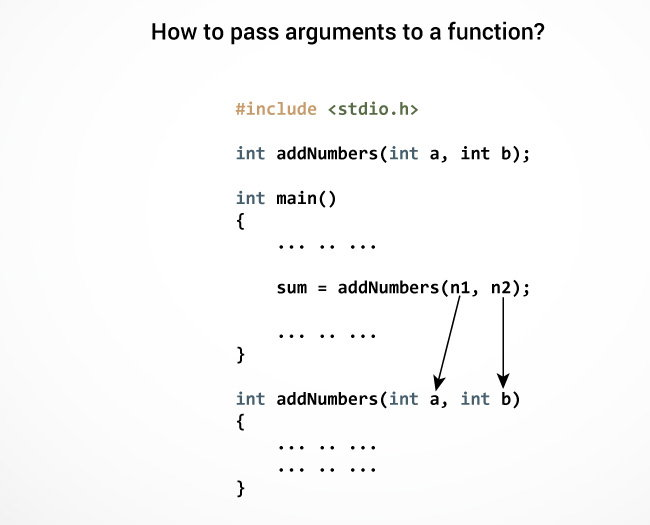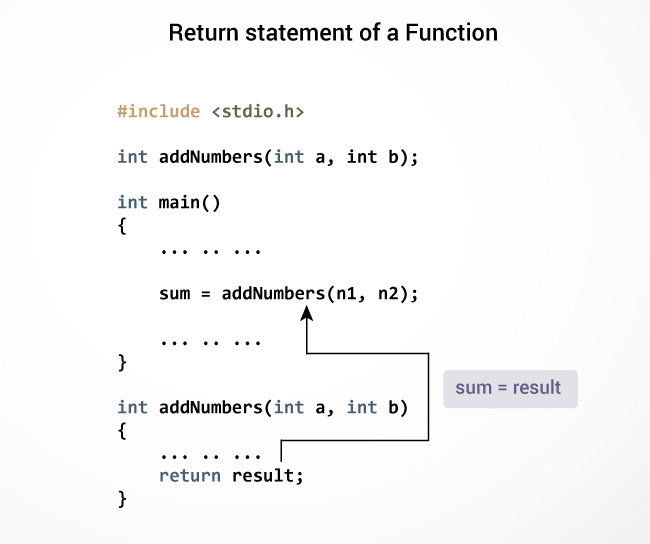C User-defined functions

# C User-defined functions

#### In this tutorial, you will learn to create user-defined functions in C programming with the help of an example.

A function is a block of code that performs a specific task.

C allows you to define functions according to your need. These functions are known as user-defined functions. For example:

Suppose, you need to create a circle and color it depending upon the radius and color. You can create two functions to solve this problem:

• `createCircle()` function
• `color()` function

## Example: User-defined function

Here is an example to add two integers. To perform this task, we have created an user-defined `addNumbers()`.

``````#include <stdio.h>
int addNumbers(int a, int b);         // function prototype

int main()
{
int n1,n2,sum;

printf("Enters two numbers: ");
scanf("%d %d",&n1,&n2);

sum = addNumbers(n1, n2);        // function call
printf("sum = %d",sum);

return 0;
}

int addNumbers(int a, int b)         // function definition
{
int result;
result = a+b;
return result;                  // return statement
}```
```

## Function prototype

A function prototype is simply the declaration of a function that specifies function's name, parameters and return type. It doesn't contain function body.

A function prototype gives information to the compiler that the function may later be used in the program.

### Syntax of function prototype

`returnType functionName(type1 argument1, type2 argument2, ...);`

In the above example, `int addNumbers(int a, int b);` is the function prototype which provides the following information to the compiler:

1. name of the function is `addNumbers()`
2. return type of the function is `int`
3. two arguments of type `int` are passed to the function

The function prototype is not needed if the user-defined function is defined before the `main()` function.

## Calling a function

Control of the program is transferred to the user-defined function by calling it.

### Syntax of function call

`functionName(argument1, argument2, ...);`

In the above example, the function call is made using `addNumbers(n1, n2);` statement inside the `main()` function.

## Function definition

Function definition contains the block of code to perform a specific task. In our example, adding two numbers and returning it.

#### Syntax of function definition

```returnType functionName(type1 argument1, type2 argument2, ...)
{
//body of the function
}
```

When a function is called, the control of the program is transferred to the function definition. And, the compiler starts executing the codes inside the body of a function.

## Passing arguments to a function

In programming, argument refers to the variable passed to the function. In the above example, two variables n1 and n2 are passed during the function call.

The parameters a and b accepts the passed arguments in the function definition. These arguments are called formal parameters of the function.The type of arguments passed to a function and the formal parameters must match, otherwise, the compiler will throw an error.

If n1 is of char type, a also should be of char type. If n2 is of float type, variable b also should be of float type.

A function can also be called without passing an argument.

## Return Statement

The return statement terminates the execution of a function and returns a value to the calling function. The program control is transferred to the calling function after the return statement.

In the above example, the value of the result variable is returned to the main function. The sum variable in the `main()` function is assigned this value.### Syntax of return statement

```return (expression);
```

For example,

```return a;
return (a+b);```

The type of value returned from the function and the return type specified in the function prototype and function definition must match.